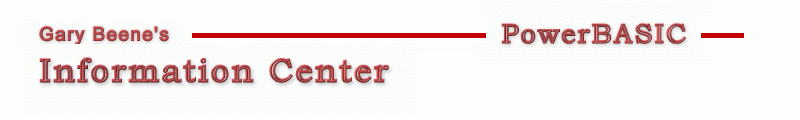Date: 03-28-2012

'Compilable Example:
'By convention, the right and bottom edges of the rectangle are normally considered exclusive.
'In other words, the pixel whose coordinates are ( right, bottom ) lies immediately outside of
'the rectangle. For example, when RECT is passed to the FillRect function, the rectangle is
'filled up to, but not including, the right column and bottom row of pixels. This structure
'is identical to the RECTL structure.
#Compiler PBWin 9, PBWin 10
#Compile EXE
#Dim All
#Include "Win32API.inc"
%IDC_GraphicA = 500
%IDC_GraphicB = 501
%IDC_Button  = 502

Global hDlg As Dword

Function PBMain() As Long
Dialog New Pixels, 0, "Gradient Fill Test",300,300,200,100, %WS_OverlappedWindow To hDlg
Control Add Graphic, hDlg, %IDC_GraphicA, "", 10,10,75,50
Control Add Graphic, hDlg, %IDC_GraphicB, "", 105,10,75,50
Control Add Button, hDlg, %IDC_Button, "Compare", 60,70,70,25
Graphic Attach hDlg, %IDC_GraphicA
Dialog Show Modal hDlg Call DlgProc
End Function

CallBack Function DlgProc() As Long
Local i,j,w,h,CT,CB As Long, r As Single, temp\$
Select Case Cb.Msg
Case %WM_InitDialog
CT = %Red  : CB = %Blue
'apply gradient color to right Graphic Control using custom GetColor function
Graphic Attach hDlg, %IDC_GraphicB, ReDraw
Control Get Client hDlg, %IDC_GraphicB To w,h
For i = 0 To w-1
For j = 0 To h-1
r = j/(h-1)
Graphic Set Pixel (i,j), GetColor(r,CT,CB)
Next j
Next i
Case %WM_Command
Select Case %IDC_Button
If Cb.CtlMsg = %BN_Clicked Then
Control Get Client hDlg, %IDC_GraphicB To w,h
For j = 0 To h-1
Graphic Attach hDlg, %IDC_GraphicA
Graphic Get Pixel (10,j) To CT
temp\$ = temp\$ + Format\$(GetRValue(CT),"000-") + Format\$(GetGValue(CT),"000-") + Format\$(GetBValue(CT),"000")
Graphic Attach hDlg, %IDC_GraphicB
Graphic Get Pixel (10,j) To CB
temp\$ = temp\$ + Format\$(GetRValue(CB),"   000-") + Format\$(GetGValue(CB),"000-") + Format\$(GetBValue(CB),"000") + \$CrLf
Next j
? temp\$
End If
End Select
End Select
End Function

Sub CreateGradientColors (clrTop As Long, clrBottom As Long)
Local hDC As Dword, w,h As Long, gRect As Gradient_Rect
Dim V(1) As TriVertex
Control Get Client hDlg, %IDC_GraphicA To w,h
Graphic Get DC To hDC

V(0).x      = 0
V(0).y      = 0
V(0).Red    = Mak(Word,0,GetRValue(clrTop))
V(0).Green  = Mak(Word,0,GetGValue(clrTop))
V(0).Blue   = Mak(Word,0,GetBValue(clrTop))
V(1).x      = w    '<---- not w-1
V(1).y      = h    '<---- not h-1
V(1).Red    = Mak(Word,0,GetRValue(clrBottom))
V(1).Green  = Mak(Word,0,GetGValue(clrBottom))
V(1).Blue   = Mak(Word,0,GetBValue(clrBottom))

gRect.UpperLeft = 0
gRect.LowerRight = 1

Graphic ReDraw
End Sub

Function GetColor(r As Single, ColorTop As Long, ColorBottom As LongAs Long
Local R1,G1,B1,R2,G2,B2 As Long
R1 = GetRValue(ColorTop)
G1 = GetGValue(ColorTop)
B1 = GetBValue(ColorTop)
R2 = GetRValue(ColorBottom)
G2 = GetGValue(ColorBottom)
B2 = GetBValue(ColorBottom)
Function = RGB( (R1 + (R2-R1)*r), (G1 + (G2-G1)*r), (B1 + (B2-B1)*r) )
End Function

'gbs_00881
'Date: 03-10-2012

created by gbSnippets
http://www.garybeene.com/sw/gbsnippets.htm